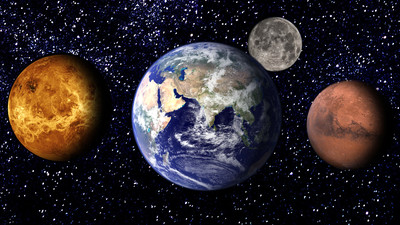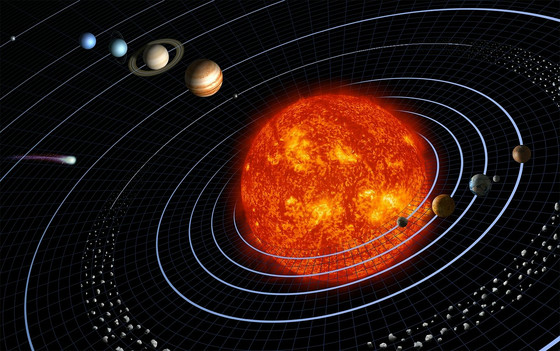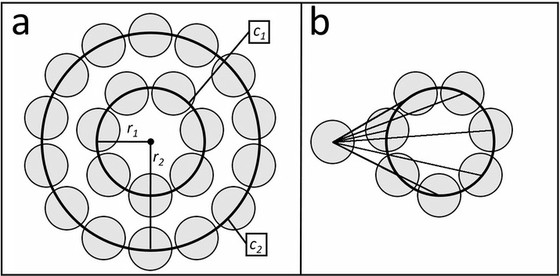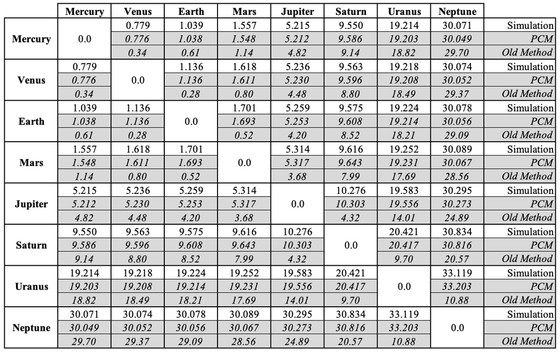# In the claim that 'Venus is not the closest planet to Earth,' which planet is closest to Earth?In the solar system, eight planets, Mercury, Venus, Earth, Mars, Jupiter, Saturn, Uranus and Neptune, revolve around the Sun. It is generally said that the planet closest to the earth where we live is Venus , and it is described as "our closest planetary neighbor (our closest neighbor)" on the NASA introduction page by NASA . Astronomers have argued that "the planet closest to Earth is not Venus," against the prevailing theory.

Venus is not Earth's closest neighbor
https://physicstoday.scitation.org/do/10.1063/PT.6.3.2090312a/full/

The distance between the planets is compared by the average distance between the planets, and in the conventional way, it is calculated by the difference between the average orbital radius (distance from the sun) of the two planets. For example, the average distance between a Venus with an average orbital radius of 0.72 AU (about 108 million km) and an earth with an average orbital radius of 1.00 AU (150 million kim) is 1.00-0.72 = 0.28 AU (about 42 million km).However, the difference in the mean revolution radius is close to the distance when the two planets are closest to each other, and the distance of 0.28 AU is not always maintained between the two planets. Therefore, three people, Tom Stockman and others who are research assistants at Los Alamos National Laboratory , are arguing against the established theory of determining which is the closest planet only by the difference in average rotation radius.

The three have proposed a new calculation method called "Point Circle Method (PCM)" in order to grasp the average distance between planets more accurately. In PCM, the orbits of each planet are assumed to be "coplanar concentric circles with mean radius". Three commented that "this assumption is not wrong in our solar system, and the eight planets have an orbital inclination of 2.6 degrees ± 2.2 degrees, and the average orbital eccentricity is 0.06 ± 0.06."

Figure a below shows the trajectories of two planets. c1 is the orbit of the inner planet with the mean orbital radius = r1, and c2 is the orbit of the outer planet with the mean orbital radius = r2. Since the planets are always moving at a constant revolution speed on the orbital circle, the probability distribution of "where on the orbits the planets are in" is considered to be uniform. Therefore, the three of them "average of the distance from any point on c2 (left circle) to all points on c1 (right circle group)" as shown in Fig. B, between the new two planets It was mathematically defined as the mean distance.As a result of the calculation, the average distance between Earth and Venus is 1.14 AU (about 170 million km), and the average distance between Mars is 1.70 AU (about 250 million km), while the average with Mercury is It turned out that the distance was 1.04 AU (about 155 million km). From this, "The closest planet to Earth is Mercury," argues Stockman.

At the same time, Stockman et al. Used a Python library called PyEphem to simulate the position of all eight planets in the solar system and compare the 10-year average measurement distance with the calculated results in PCM. You can see how the simulation is moving from around 6 minutes and 40 seconds of the following movie:

Mercury is the closest planet to all seven other planets-YouTube

The following table shows the average distance between each planet from the top by "calculation by simulation", "calculation by PCM" and "calculation by the conventional method". It is clear that the distance derived by simulation and the distance calculated by PCM are almost the same.Furthermore, three people have developed the reasoning from this result that "the smaller the orbit radius of the inner object, the shorter the average distance between the concentrically moving objects". Although not completely proven, based on this inference, the innermost orbiting Mercury is the closest planet to all other planets.

According to three people, PCM can be used to quickly estimate the average distance of any orbiting object, for example, to help quickly verify satellite networks where signal strength decreases in proportion to the square of distance And. "We found that at least Venus is not our closest neighbor, and that Mercury is everyone's neighbor," Stockman argued.

in Science, Posted by log1i_yk